Parametric equation

of a set of points in a space

The specification of the points of the set or of their coordinates by the values of functions of certain variables called parameters.

The parametric representation of a straight line in the-dimensional vector spacehas the form(1)

whereandare fixed vectors:is the initial vector and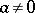is a directed vector parallel to the line. If a basis inis given and if the coordinates of the vectorsandare denoted byand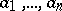, respectively, then (1) in coordinate form becomesThe parametric representation of an-dimensional affine subspace inhas the form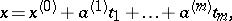(2)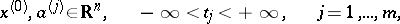where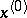is the initial vector corresponding to the value 0 of the parameters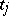and theform a linearly independent system ofvectors parallel to the affine subspace in question. In coordinate form (2) becomes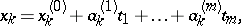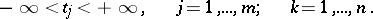The parametric representation of an-dimensional surface inhas the form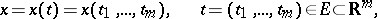(3)

whereis, for example, the closure of a certain domain in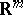and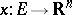is a mapping of a certain class: continuous, differentiable, continuously differentiable, twice differentiable, etc.; accordingly, the-dimensional surface is also called continuous, differentiable, etc. (The rank of the Jacobian matrix is supposed to be.) In the case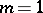the setis an interval,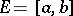, and (3) becomes the parametric representation of a curve: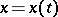,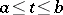, in. For example,,,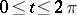, is a parametric representation in the plane of the circle of radius 1 with centre at the coordinate origin.

For the seton which the parametric representation is given one sometimes takes instead of the closure of an-dimensional domain a subset ofof another kind.

A parametric equation or parametric representation for an-dimensional surfacein(or) need not be of dimension. I.e. any surjective mapping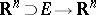with as image (an open piece of) the surfaceis a (local) parametric representation of.
A chart is a local parametric representation (equation) forof dimension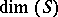. Given a chart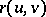of a surfacein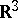, the curves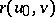,fixed,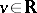, and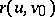,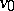fixed,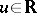, are called parametric curves.